# Denominator 2

Denominator of a fraction is 5 and numerator is 7. Write the fraction .

f =  1.4

### Step-by-step explanation:

$f=\frac{7}{5}=\frac{7}{5}=1\frac{2}{5}=1.4$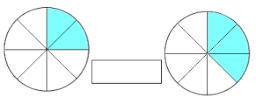Did you find an error or inaccuracy? Feel free to write us. Thank you!Tips to related online calculators
Need help to calculate sum, simplify or multiply fractions? Try our fraction calculator.

## Related math problems and questions:

• FractionFraction ? write as fraction a/b, a, b is integers numerator/denominator.
• Division by zero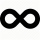Fraction 5 by 2. if 3 is added to numerator and 2 is subtracted from the denominator then the new fraction is:
• Guess a fractionTom was asked to guess a fraction. The sum of 1/2 the numerator and 1/3 of its denominator is 30. If Tom subtracts 36 from its denominator, the fraction becomes 1/3. What is the fraction that Tom was asked to guess? (Leave your answer in simplest form)
• When 5When 5 is subtracted from both my numerator and denominator I become 3/4. What fraction i am?
• A fractionI think of a fraction, if we increase its numerator and denominator by one, the value of the fraction increases by one tenth, what fraction do I think?
• Over fractionSolve equation: p over 5 equals fraction numerator 7 p over denominator 6 end fraction plus 5
• Denominator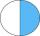Calculate the missing denominator x: (264)/(96) = (11)/(x)
• Mixed numbersRewrite mixed numbers, so the fractions have the same denominator: 5 1/5 - 2 2/3
• Write 2Write 791 thousandths as fraction in expanded form.
• Fraction and a decimalWrite as a fraction and a decimal. One and two plus three and five hundredths
• Fraction to decimalWrite the fraction 3/22 as a decimal.
• JerryJerry has 3/4 of a pizza. He needs to share it with 6 friends. What fraction of the pizza will each friend get? Only write the fraction
• Find twoFind two consecutive natural numbers whose product is 1 larger than their sum. Searched numbers expressed by a fraction whose numerator is the difference between these numbers and the denominator is their sum.
• Write 3Write a real world problem involving the multiplication of a fraction and a whole number with a product that is between 8 and 10 then solve the problem
• Difference of two fractions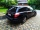What is the difference between 1/2 and 1/6? (Write the answer as a fraction in lowest terms. )
• Carrie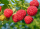Carrie picked 2/5 of the raspberries from the garden, and Robin picked some too.  When they were finished, 1/3 of the raspberries still needed to be picked.  What fraction of the raspberries did Robin pick? Use pictures, numbers or words and write your fi
• Divide 13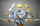Divide. Simplify your answer and write as an improper fraction or whole number. 14÷8/3